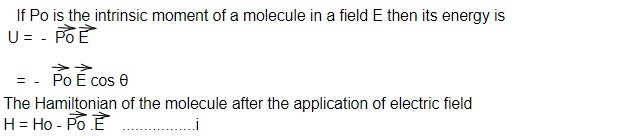# Polarisability per molecule in terms of Langevin's function

In polar substance, the permanent dipoles are random oriented due to the thermal agitation. So the net dipole moment is zero. When an external field is applied, the dipoles align themselves in the direction of applied field.

In statistical mechanics, under the condition of thermal equilibrium, the probability that one molecule has energy U is proportional towhere Ho is the Hamiltonian in the absence of electric field. The energy of the molecules given by eq i depends upon the orientation of the molecules with respect to the field. The contribution of each molecules to the total dipole moment would be Pocosθ which we have to sum over all the molecules which are agitated.

Then the average dipole moment is given by

< P mol > =Po (cot hx - 1/x)

< P mol > = Po L(x) where L (x) = (cot hx - 1/x) is called Langevin function.

For higher temperature

x = PoE/ KBT << 1, then the Langevin function becomes

cot hx = 1/x 1/x + x/3 +................-1/x

< P mol > = Po x/3

We have, < Pmol > ∝ E

or, < Pmol > = α E

α = < Pmol > / E

where α = molecular polarisability

This note is taken from Statistical Mechanics, MSC physics, Nepal.

This note is a part of the Physics Repository.

Previous topic: Langevin's theory of Paramagnetism